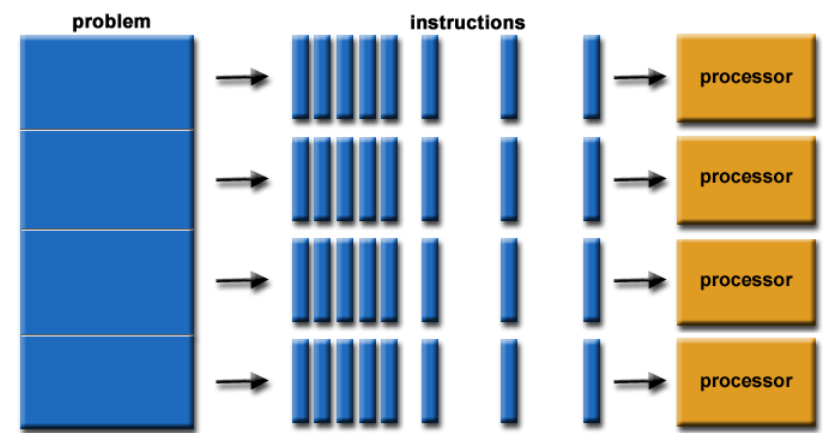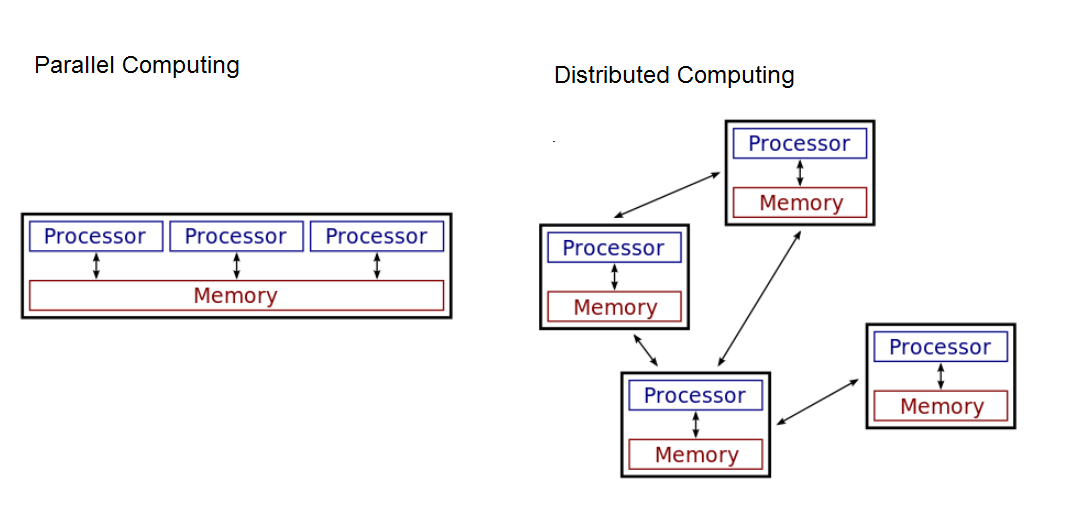# 5.9. Parallel Computing¶

## 5.9.1. Introduction and Goals¶

### Parallel Computing

For hard problems that take a long time to solve, we can sometimes speed up the algorithm by using multiple processors or computers. We can split the workload and compute the parts of the solution in parallel.
• Sequential computing is a computational model in which operations are performed in order, one at a time on one processor or computer.
• Parallel computing is a computational model where a problem or program is broken into multiple smaller sequential computing operations some of which are performed simultaneously in parallel. This is usually on one computer with multiple processors, but it could also use multiple computers.
• Distributed computing is a computational model in which multiple networked computers are used to run a program. An algorithm can be both parallel and distributed.Learning Objectives: I will learn to
• compare sequential and parallel computing solutions
• determine the efficiencies of sequential and parallel computing solutions
Language Objectives: I will be able to
• use target vocabulary, such as distributed computing and speedup while describing the benefits and challenges of parallel computing with the support of concept definitions and vocabulary notes from this lesson

## 5.9.2. Learning Activities¶

### Searching POGIL Activity

Each group of four should be given a deck of cards (or use PlayingCards.io) Have one team member time the following tasks:
1. Sequential Algorithm: Have one team member search through the deck of cards one card at a time for the Queen of Hearts (using a linear search) while the rest of the team times them. How long did it take? If you find the card right away, put the Queen of Hearts near the bottom of the deck, and time the search for it again to record the worst case longest time it takes to find a card sequentially.
2. Parallel Algorithm: Start the timer. Divide the group’s deck of cards into four roughly equal stacks of cards and give each team member one stack. And have each team member search through their stack of cards one card at a time in parallel looking for the Queen of Hearts. Yell out "found it" when someone in the group finds it and stop the clock. How long did it take?
3. Speedup: We can compute the speedup of a parallel solution by dividing the time it took to do the task sequentially by the time it took to complete the task in parallel. What is the speedup of your search algorithm?

We can compare the efficiency of sequential vs. parallel solutions by comparing the time it takes them to perform the same task. A sequential solution takes as long as the sum of all of its steps. In the card activity, in the worst case, you would need to look through 52 cards with the sequential algorithm to find a particular card. A parallel computing solution takes as long as its sequential tasks (for example, splitting up the deck of cards into 4 stacks) plus the longest of its parallel tasks (for example, finding the card in parallel). In the parallel algorithm card activity, the 52 cards were divided into 4 stacks, and the 4 team members each looked through around 13 cards in the worst case to find the card in parallel.

The speedup of a parallel solution is measured in the time it took to complete the task sequentially divided by the time it took to complete the task when done in parallel. The speedup for the card activity could be close to four times as fast with the parallel algorithm.

### Benefits and Challenges in Parallel Computing

Solutions that use parallel computing can scale up which means that they can get faster as we add more processors. However, there is a limit to this speed up. Parallel computing consists of a parallel portion and a sequential portion. The sequential portion is usually before and after the parallel part to divide the workload and combine the results. The time taken is the sum of the time taken in the sequential and parallel parts. This means the efficiency of the solution is limited by the sequential portion, at some point, adding parallel portions will no longer meaningfully increase efficiency.

### Sorting POGIL Activity

Each group of four should be given a deck of cards. Have one team member time the following tasks.
1. Parallel Sorting with 2 processors: One team member should start the timer. Divide the group’s deck of cards into two roughly equal stacks of cards and give two team members each stack. Have each of the two team members sort their stack of cards in parallel. When they are done, have another team member merge together the two stacks into one sorted deck of cards. Stop the timer. How long did it take?
2. Parallel Algorithm with 4 processors: Mix up the cards. Start the timer. Divide the group’s deck of cards into four roughly equal stacks of cards and give each team member one stack. Have each team member sort their stack. Then have one team member merge together the four sorted stacks to make one sorted stack. Stop the timer. How long did it take?
3. Speedup: Was it faster to use four processors instead of two? How was the speedup affected by the sequential part of the algorithm which was the merge?
4. Reflection: What are the benefits and challenges of parallel computing?

### Distributed Computing

In Distributed Computing, multiple networked computers are used to solve a problem. Distributed computing allows problems to be solved that could not be solved on a single computer because of the required long processing time or large storage needs. And it allows much larger problems to be solved quicker than they could be solved using a single computer.Watch the following video for distributed computing in practice at Folding@Home where you can donate distributed computer time to solve real world problems. They also have a new initiative to help with COVID-19 research.

## 5.9.3. Summary¶

In this lesson, you learned how to:

## 5.9.4. Self-Check¶

Here is a table of some of the technical terms discussed in this lesson. Hover over the terms to review the definitions.

 sequential computing parallel computing distributed computing speedup

## 5.9.5. Reflection: For Your Portfolio¶

Answer the following portfolio reflection questions as directed by your instructor. Questions are also available in this Google Doc where you may use File/Make a Copy to make your own editable copy.